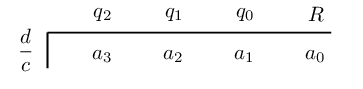Home Practice
For learners and parents For teachers and schools
Textbooks
Full catalogue
Pricing SupportLog in

We think you are located in United States. Is this correct?

# 5.6 Summary

## 5.6 Summary (EMCGY)

 Terminology: Expression A term or group of terms consisting of numbers, variables and the basic operators ($$+, -, \times, \div$$). Univariate expression An expression containing only one variable. Root/Zero A root, also referred to as the “zero”, of an equation is the value of $$x$$ such that $$f(x)=0$$ is satisfied. Polynomial An expression that involves one or more variables having different powers and coefficients. $$a_{n}x^{n} + \ldots + a_2x^{2} + a_{1}x + a_{0}, \text{ where } n \in \mathbb{N}_0$$ Monomial A polynomial with one term. For example, $$7a^{2}b \text{ or } 15xyz^{2}$$. Binomial A polynomial that has two terms. For example, $$2x + 5z \text{ or } 26 - g^{2}k$$. Trinomial A polynomial that has three terms. For example, $$a - b + c \text{ or } 4x^2 + 17xy - y^3$$. Degree/Order The degree, also called the order, of a univariate polynomial is the value of the highest exponent in the polynomial. For example, $$7p - 12p^2 + 3p^5 + 8$$ has a degree of $$\text{5}$$.
• Quadratic formula: $$x = \frac{-b±\sqrt{{b}^{2}-4ac}}{2a}$$

• Remainder theorem: a polynomial $$p(x)$$ divided by $$cx - d$$ gives a remainder of $$p\left(\dfrac{d}{c}\right)$$.

• Factor theorem: if the polynomial $$p(x)$$ is divided by $$cx - d$$ and the remainder, $$p \left( \frac{d}{c} \right)$$, is equal to zero, then $$cx - d$$ is a factor of $$p(x)$$.

• Converse of the factor theorem: if $$cx - d$$ is a factor of $$p(x)$$, then $$p \left( \frac{d}{c} \right) = 0$$.

• Synthetic division:We determine the coefficients of the quotient by calculating:

\begin{align*} q_{2} &= a_{3} + \left( q_{3} \times \frac{d}{c} \right) \\ &= a_{3} \quad \text{ (since } q_{3} = 0) \\ q_{1} &= a_{2} + \left( q_{2} \times \frac{d}{c} \right) \\ q_{0} &= a_{1} + \left( q_{1} \times \frac{d}{c} \right) \\ R &= a_{0} + \left( q_{0} \times \frac{d}{c} \right) \end{align*}
temp text